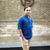# Find duplicate by comparing two fields.

1124
9
03-23-2017 09:43 AMRegular Contributor

I need to find duplicates by comparing to fields. I am not sure how to accomplish this so any help would be appreciated.

I have two fields that i need to compare, Account and Acres1 fields. if there is not duplicate then put a "0" in the duplicate field for that record.

The end scenario i need something like this.

``````Account    Acres   Duplicate
D54963     12.43    0
D54963     12.43    1 (duplicate)
D54963     12.43    2 (duplicate)

D45879     26.32    0 (no duplicate)
D45879     17.32    0 (no duplicate)

D36540     17.51    0
D36540     17.51    1 (duplicate)
D36540     17.51    2 (duplicate)
D36540     17.51    3‍‍‍‍‍‍‍‍‍ (duplicate)‍‍‍‍‍‍‍‍‍‍‍‍‍‍‍‍‍‍‍‍‍‍‍‍‍‍‍‍‍‍‍‍‍‍``````

I have the following code but it only checks one field (Account) and it add a number to all attributes if there is a double not exactly what i need.

``````import arcpy

infeature ="C:/Temp/Test_FINAL_Erase"
field_in ="Account"
field_out ="COUNT_"+field_in

#create the field for the count values

#creating the list with all the values in the field, including duplicates
lista=[]
cursor1=arcpy.SearchCursor(infeature)
for row in cursor1:
i=row.getValue(field_in)
lista.append(i)
del cursor1, row

#updating the count field with the number on occurrences of field_in values
#in the previously created list
cursor2=arcpy.UpdateCursor(infeature)
for row in cursor2:
i=row.getValue(field_in)
occ=lista.count(i)
row.setValue(field_out,occ)
cursor2.updateRow(row)
del cursor2, row
print ("Done.")
‍‍‍‍‍‍‍‍‍‍‍‍‍‍‍‍‍‍‍‍‍‍‍‍‍‍‍‍‍‍‍‍‍‍‍‍‍‍‍‍‍‍‍‍‍‍‍‍‍‍‍‍‍‍‍‍‍``````
1 Solution

Accepted SolutionsbyMVP Esteemed Contributor

Does the following work:

``````from collections import defaultdict

fc = # path to feature class

dups = defaultdict(int)
with arcpy.da.UpdateCursor(
fc,
["Account", "Acres", "Duplicate"],
sql_clause=(None, "ORDER BY Account, Acres")
) as cur:
for acct, acres, _ in cur:
dups[(acct, acres)] += 1
cnt = dups[(acct, acres)] - 1
cur.updateRow([acct, acres, cnt])‍‍‍‍‍‍‍‍‍‍‍‍‍‍``````
9 RepliesbyMVP Esteemed Contributor

Is it possible for a given account number to have multiple, different acre values?  For example, could D54963 have 12.43 acres and some other acre value?Regular Contributor

Joshua yes that is a possibility. Sorry i should have mentioned that.byMVP Esteemed Contributor

Not an answer to your question, but sometimes the floating values might to be difficult to select using "=" operator, because

ArcGIS might interpret a floating value of 12.43 as 12.429999 or 12.430001.

Think LocationbyMVP Frequent Contributor
``````fc = r'H:\Documents\ArcGIS\Default.gdb\MyFeatureClass'
fields= ["OBJECTID", "Field1", "Field2"]
with arcpy.da.SearchCursor(fc, fields) as cursor:
for row in cursor:
if row==row:
sql = "OBJECTID=" + str(row)
with arcpy.da.UpdateCursor(fc, "Field3", sql) as ucur:
for urow in ucur:
urow=0
ucur.updateRow(urow)‍‍‍‍‍‍‍‍‍‍``````Regular Contributor

Actually i thought it was your code that that Count_Acres1 but it wasn't. The code you posted ran but did not don't do anything.

What i have

``````# Import the arcpy module
import arcpy

fc = r'C:Temp/Test_Erase'
fields= ["OBJECTID", "ACCOUNT", "Acres1"]
with arcpy.da.SearchCursor(fc, fields) as cursor:
for row in cursor:
if row==row:
sql = "OBJECTID=" + str(row)
with arcpy.da.UpdateCursor(fc, "Count", sql) as ucur:
for urow in ucur:
urow=0
ucur.updateRow(urow)
print ("Done.")‍‍‍‍‍‍‍‍‍‍‍‍‍‍‍``````byMVP Regular Contributor

In your code in line 11:

``````   with arcpy.da.UpdateCursor(fc, "Count", sql) as ucur:
‍‍‍``````

"Count" is an SQL reserved word and should not be used as a field/column name.byMVP Frequent Contributor

Not a complete solution but might be something to look into.  numpy and pandas have much better grouping functions.  Challenge is to get the result back into your arcpy.da cursor and update the desired column.

``````nparr = arcpy.da.FeatureClassToNumPyArray(fc,["Account", "Acres"])
pdarr = pd.DataFrame(nparr)
counts = pdarr.groupby(["Account", "Acres"]).size()
pddups = pd.DataFrame(counts, columns = ['size'])
pddups = pddups[pddups.size>1]
print pddups‍‍‍‍‍‍``````byMVP Esteemed Contributor

Does the following work:

``````from collections import defaultdict

fc = # path to feature class

dups = defaultdict(int)
with arcpy.da.UpdateCursor(
fc,
["Account", "Acres", "Duplicate"],
sql_clause=(None, "ORDER BY Account, Acres")
) as cur:
for acct, acres, _ in cur:
dups[(acct, acres)] += 1
cnt = dups[(acct, acres)] - 1
cur.updateRow([acct, acres, cnt])‍‍‍‍‍‍‍‍‍‍‍‍‍‍``````Regular Contributor

Joshua,

Ya i think it worked. Thank you, you saved me a lot of time!

Thanks!